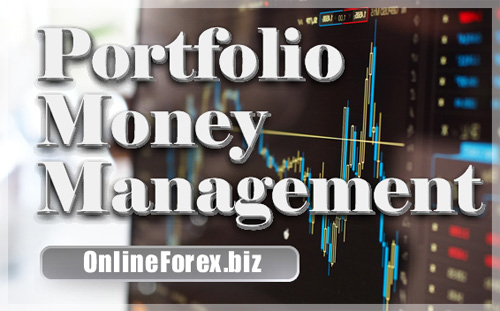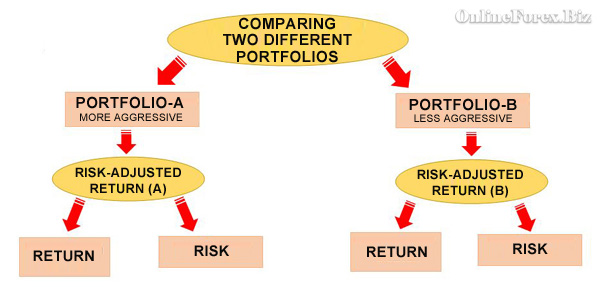# Portfolio ManagementThis article presents three basic investment concepts and a series of significant portfolio management ratios.

Three Basic Investment Concepts

• risk-free rate
• standard deviation
• max drawdown

(i) Risk-Free Rate

The 'Risk-Free Rate' refers to the annual return that an investor can secure without taking any market risk. Usually the 'Risk-Free Rate' is calculated based on the annualized interest paid by holding a three (3) month treasury bill. Any investment offering lower returns than this guaranteed rate is considered an unacceptable investment.

• In the EU, the 3-month Euribor is used
• In the USA, the 3-month US Treasury bill

(ii) Standard Deviation

Standard deviation (SD) is a statistical tool that estimates the amount of variation in a set of values. A low standard deviation indicates variations close to the mean value, while conversely, a high standard deviation indicates a large variation from the mean value. This average value is also called the expected value.

But why is it important for a portfolio to have a low standard deviation? The reason is that a low standard deviation ensures that a portfolio's positive performance is consistent over time, and thus can easily be repeated in the future.

Using standard deviation, investors can normalize a portfolio's historical returns, thus better evaluating them.

• Standard deviation can be used to normalize the historical returns of individual assets or even entire investment portfolios
• The smaller the estimated standard deviation of a portfolio the better, as this means that the portfolio's returns are consistent and predictable over time
• A portfolio with a low standard deviation is considered better than a portfolio with a high standard deviation
• The standard deviation is usually calculated on a monthly or yearly basis

(iii) MaxDrawdown

The MaxDrawdown calculates the maximum historical loss of a portfolio from its maximum value. The index positively evaluates portfolios that show limited losses over time.

• The lower the 'MaxDrawdown', the better for an investment portfolio
• When comparing identical investment portfolios, the portfolio showing the lowest 'MaxDrawdown' ratio is considered the best
• The longer the analysis period, the higher the 'Maximum Drawdown' indicator will be measured

Portfolio Management Ratios

Portfolio management aims to achieve the highest possible return while accepting the lowest possible risk:

(i) Maximizing return

(ii) Minimizing the chances and consequences of a loss

But if return and risk are the two variables that determine the success of capital management, combining these two elements into a single metric could make portfolio management much easier. This combination is called risk-adjusted portfolio return and is commonly used by professional market managers.

Based on the unification of performance and risk in a single measurement, it becomes possible to compare portfolios that historically display different qualitative characteristics, as shown in the graph below.

Graph: The integration of return and risk helps to compare portfolios with different qualitative characteristicsThe integration of return and risk into a single metric is essential both for evaluating personal portfolios and for evaluating and comparing mutual funds, ETFs, etc. At the same time, by combining risk and return, it is realized that the portfolio that offered the highest return in the past is not necessarily the best portfolio to invest in in the future.

Here are some of the most important ratios of a portfolio's risk-adjusted return:

• Sharpe ratio
• Sortino index
• Treynor index
• Jensen Measure or Jensen's Alpha
• Calmar index
• MAR index
• Omega index
• Information Ratio

(1) Sharpe ratio

■ Sharpe Ratio = {(P - RFR) / SD(P)}

where:

□ P = Expected return

□ RFR = Risk-Free Rate

□ SD(P) = Standard deviation of a portfolio returns

(2) Sortino index

■ Sortino Ratio = (P - T) / SD(dP)

where:

□ P = Average annual return

□ T = Targeted-return

□ SD(dP) = Standard deviation of negative annual returns

(3) Treynor index

■ Treynor = (P - RFR) / b

where:

P = Portfolio performance

RFR = Risk-Free Rate

β = measures the volatility of a portfolio relative to the general market

(4) Jensen's Alpha or Jensen's Measure

■ Jensen's Alpha = P - {RFR + β * (P(m) - RFR)}

where:

□ P = The return on the portfolio

□ RFR = Risk-Free Rate

□ β = Beta coefficient

□ P(m) = The market return

(5) Calmar index

■ Calmar Ratio = (P - RFR) / MaxDrawdown

where:

□ P = Average annual portfolio return

□ RFR = Risk-Free Rate

□ MaxDrawdown (as above)

(6) MAR index

■ MAR Ratio = CAGR / MaxDrawdown

where:

□ CAGR = Compound Annual Growth Rate

□ MaxDrawdown (as above)

(7) Omega index

■ Omega Ratio = {Σ(Winning) - Benchmarking} / {Σ(Benchmarking) - Losing}

where:

□ Benchmarking = a reference performance

(8) Information Ratio

■ Information Ratio = {P - P(b)} / SD

where:

□ P = Portfolio performance

□ P(b) = Performance of a benchmark

□ SD = Standard deviation

Conclusions

• The risk of losing part of your capital is just as significant (if not more significant) than the expectation of achieving a high return
• The portfolio that has achieved the highest return in the past is not necessarily the best portfolio to invest in. The risk taken to achieve this return must be factored in
• Combining risk and return into a single metric forms the risk-adjusted return. Based on this metric, the actual returns of portfolios with different qualitative characteristics can be compared
• There are several indicators for evaluating a portfolio's returns, with Sharpe being the most popular of them
• The Risk-Free Rate refers to the annual return that the market offers without any risk. Any investment expected to offer lower returns than this percentage is considered an unacceptable investment
• The 'MaxDrawdown' indicator measures the maximum loss of a portfolio from its maximum value (the lower the better)
• The standard deviation assesses whether a portfolio's returns are consistent and predictable over time. A portfolio that exhibits a low standard deviation is considered better than a similar portfolio that exhibits a high standard deviation

Portfolio Management

G.P. for OnlineForex.biz (c)